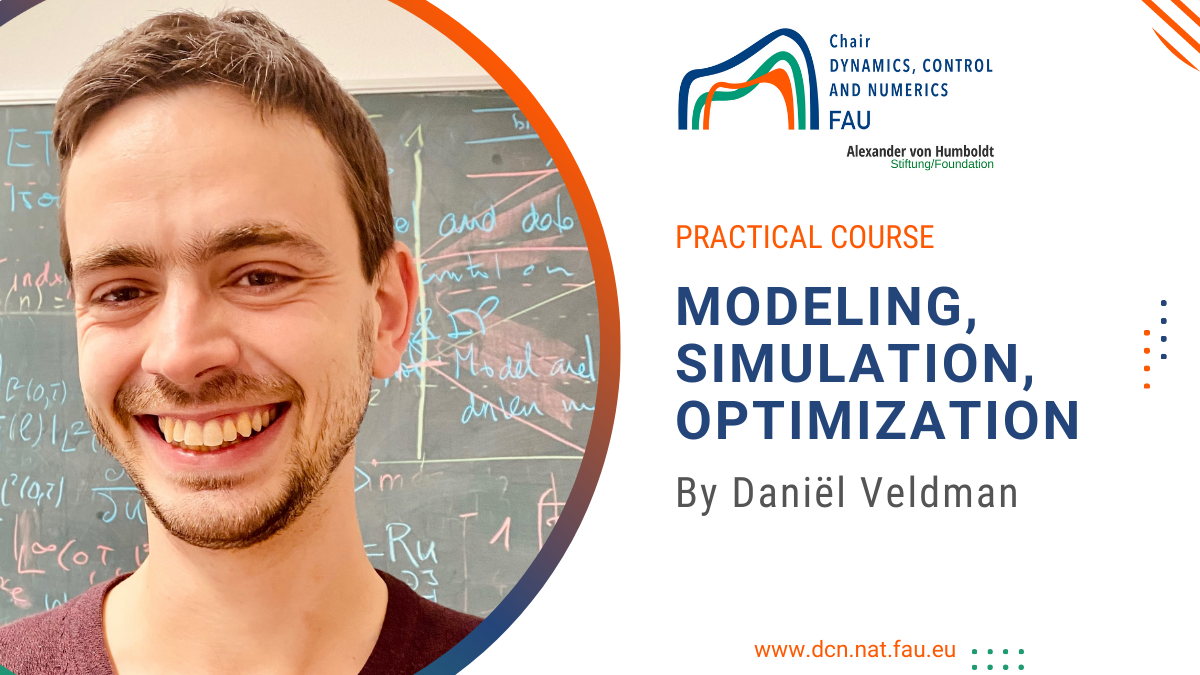# Practical course: Modeling, simulation, optimization#### Practical course: Modeling, simulation, optimization

FAU DCN-AvH. Friedrich-Alexander Universität Erlangen-Nürnberg (Germany)
Period: Summer semester 2021

This course provides a practical introduction to some of the most commonly used discretization methods for PDEs (finite differences and finite elements) and their implementation in MATLAB. It also covers some of the basics of gradient-based optimization focused on the numerical solution of (PDE-constrained) optimal control problems. Some basic programming skills in MATLAB or a similar programming language is recommended but not required.

The course material is structured into 12 weeks. For each week, you can find the lecture slides (pdf), a related MATLAB exercise (pdf), and a starting point for the MATLAB code (zip). The 12 lectures are structured into three parts of 4 lectures each. The different parts can be studied largely independent, but sometimes rely on previously covered topics. In particular the material on time discretization from lecture 3 in part 1 also plays an important role in the other parts. For each of the three part there is a bonus exercise. The bonus questions are significantly more advanced than the other exercises and they do not always come with a starting point for the MATLAB code.

#### Part I. Finite Differences

• Lecture 1: Finite differences for the 1-D Poisson equation
o Slides
o Exercise
o MATLAB files
• Lecture 2: Finite differences for the 2-D Poisson equation
o Slides
o Exercise
o MATLAB files
• Lecture 3: Time-discretization
o Slides
o Exercise
o MATLAB files
• Lecture 4: Von Neumann stability analysis and advection-Diffusion equations
o Slides
o Exercise
o MATLAB files

#### Part II. Finite Elements

• Lecture 5: Finite elements in 1-D
o Slides
o Exercise
o MATLAB files
• Lecture 6: Finite elements in 2-D
o Slides
o Exercise
o MATLAB files
• Lecture 7: 2-D elasticity
o Slides
o Exercise
o MATLAB files
• Lecture 8: Beam models
o Slides
o Exercise
o MATLAB files

#### Part III. Optimization

• Lecture 9: Optimization and gradient descent
o Slides
o Exercise
o MATLAB files
• Lecture 10: Hessians and step size selection
o Slides
o Exercise
o MATLAB files
• Lecture 11: Dynamic optimal control
o Slides
o Exercise
o MATLAB files
• Lecture 12: Control of neural ODEs
o Slides
o Exercise
o MATLAB files